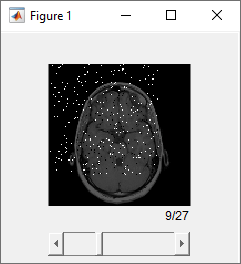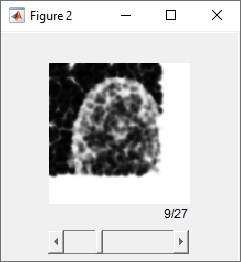# multissim3

Multiscale structural similarity (MS-SSIM) index for volume quality

## Syntax

``score = multissim3(V,Vref)``
``score = multissim3(V,Vref,Name,Value)``
``[score,qualityMaps] = multissim3(___)``

## Description

example

````score = multissim3(V,Vref)` calculates the multiscale structural similarity (MS-SSIM) index, `score`, for volume `V`, using `Vref` as the reference volume. A value closer to 1 indicates better quality and a value closer to 0 indicates poorer quality.The 3-D MS-SSIM operation is defined for grayscale volumes. For inputs with more than three dimensions, `multissim3` treats each element of higher dimensions as separate 3-D grayscale volumes. `multissim3` treats 2-D RGB images as 3-D grayscale volumes. To calculate the MS-SSIM of color channels in an RGB image, use the `multissim` function.```

example

````score = multissim3(V,Vref,Name,Value)` controls aspects of the computation using one or more name-value arguments. For example, specify the number of scales using the `'NumScales'` argument.```

example

````[score,qualityMaps] = multissim3(___)` also returns the local MS-SSIM index value for each voxel in `V`, and each of the scaled versions of `V`. The `qualityMaps` output is a cell array containing maps for each of the scaled versions of `V`, with each quality map the same size as the corresponding scaled version.```

## Examples

collapse all

Load a 3-D volume into the workspace.

```load mri D Vref = squeeze(D);```

Create a noisy version of the original volume for quality measurement comparison purposes.

`V = imnoise(Vref,'salt & pepper',0.05);`

Calculate the MS-SSIM index that measures the quality of the input volume compared to the reference volume.

`score = multissim3(V,Vref)`
```score = single 0.7261 ```

Load a volume into the workspace. This volume will be the reference volume. Create a copy of the reference volume.

```load mri D Vref = squeeze(D); V = Vref;```

Add noise to a localized part of the volume for quality comparison purposes.

```V(1:100,1:100,1:10) = imnoise(Vref(1:100,1:100,1:10),'salt & pepper',0.05); figure sliceViewer(V);```Calculate the MS-SSIM index for the volumes and retrieve the local structural similarity maps. The `multissim3` function returns `qualitymaps`, a cell array containing a local structural similarity map for each of the scaled versions of the volume. In the quality map, the value 1 indicates the highest quality.

```[score, qualitymaps] = multissim3(V,Vref); figure sliceViewer(V);```Load a volume into the workspace.

```load mri D Vref = squeeze(D);```

Create a noisy version of the volume for quality measurement comparison purposes.

`V = imnoise(Vref,'salt & pepper',0.05);`

Calculate the MS-SSIM index for the noisy volume, using the original volume as the reference. Specify how much to weigh the local MS-SSIM index calculations for each scaled volume using the `'ScaleWeights'` argument. The example uses the weights defined in the article by Wang, Simoncelli, and Bovik.

`score = multissim3(V,Vref,'ScaleWeights',[0.0448,0.2856,0.3001,0.2363,0.1333]);`

Read a volumetric image into the workspace.

```VRef = load("mristack.mat"); VRef = im2single(VRef.mristack);```

Simulate a batch of six images by replicating the image along the fourth dimension.

`VRefBatch = repmat(VRef,[1 1 1 6]);`

Create a version of the image stack with added salt and pepper noise.

`VNoisyBatch = imnoise(VRefBatch,"salt & pepper");`

Calculate the MS-SSIM of each volume in the stack.

`score = multissim3(VNoisyBatch,VRefBatch)`
```score = 1x1x1x6 single array score(:,:,1,1) = 0.8341 score(:,:,1,2) = 0.8347 score(:,:,1,3) = 0.8337 score(:,:,1,4) = 0.8333 score(:,:,1,5) = 0.8348 score(:,:,1,6) = 0.8343 ```

Read a volumetric image into the workspace.

```VRef = load("mristack.mat"); VRef = im2single(VRef.mristack);```

Create a copy of the batch of images, adding salt and pepper noise.

`Vnoisy = imnoise(VRef,"salt & pepper");`

Create unformatted `dlarray` objects for the original and noisy batch of images.

```dlref = dlarray(VRef); dlnoisy = dlarray(Vnoisy);```

Calculate the MS-SSIM score of the noisy data with respect to the original data.

`score = multissim3(dlnoisy,dlref)`
```score = 1x1 single dlarray 0.8341 ```

## Input Arguments

collapse all

Input volume, specified as a numeric array of three or more dimensions or a `dlarray` (Deep Learning Toolbox) object. Formatted `dlarray` objects cannot include more than one channel label, more than one batch label, and more than three spatial labels.

Data Types: `single` | `double` | `int16` | `uint8` | `uint16`

Reference volume, specified as a numeric array of three or more dimensions or a `dlarray` (Deep Learning Toolbox) object. Formatted `dlarray` objects cannot include more than one channel label, more than one batch label, and more than three spatial labels. The reference volume must be of the same size and data type as the input volume, `V`.

Data Types: `single` | `double` | `int16` | `uint8` | `uint16`

### Name-Value Arguments

Specify optional pairs of arguments as `Name1=Value1,...,NameN=ValueN`, where `Name` is the argument name and `Value` is the corresponding value. Name-value arguments must appear after other arguments, but the order of the pairs does not matter.

Before R2021a, use commas to separate each name and value, and enclose `Name` in quotes.

Example: `score = multissim3(V,Vref,'NumScales',3);`

Number of scales used to calculate MS-SSIM, specified as the comma-separated pair consisting of `'NumScales'` and a positive integer. Setting `'NumScales'` to `1` is equivalent to the use of the `ssim` function with the `'Exponents'` name-value pair argument set to ```[1 1 1]```. The size of the input volume limits the number of scales. The `multissim3` function scales the volume (`NumScales` - 1) times, downsampling the volume by a factor of 2 with each scaling.

Data Types: `single` | `double` | `int8` | `int16` | `int32` | `int64` | `uint8` | `uint16` | `uint32` | `uint64`

Relative values across the scales, specified as the comma-separated pair consisting of `'ScaleWeights'` and a vector of positive numbers. The length of the vector is equal to the number of scales, because each element corresponds to one of the scaled versions of the original volume. The `multissim3` function normalizes the values to 1. By default, the scale weights equal `fspecial``('gaussian',[1,numScales],1)`. The `multissim3` function uses a Gaussian distribution as the default because the human visual sensitivity peaks at middle frequencies and decreases in both directions. For an example of setting `'ScaleWeights'`, see Calculate MS-SSIM Specifying Weights for Each Scaled Volume.

Data Types: `single` | `double` | `int8` | `int16` | `int32` | `int64` | `uint8` | `uint16` | `uint32` | `uint64`

Standard deviation of the isotropic Gaussian function, specified as the comma-separated pair consisting of `'Sigma'` and a positive number. This value specifies the weighting of the neighborhood voxels around a voxel for estimating local statistics. The `multissim3` function uses this weighting to avoid blocking artifacts in estimating local statistics.

Data Types: `single` | `double` | `int8` | `int16` | `int32` | `int64` | `uint8` | `uint16` | `uint32` | `uint64`

Dynamic range of the input volume, specified as a positive number. The default value of `DynamicRange` depends on the data type of volume `V`, and is calculated as `diff(getrangefromclass(V))`. For example, the default dynamic range is `255` for volumes of data type `uint8`, and the default is `1` for volumes of data type `double` or `single` with voxel values in the range [0, 1].

Data Types: `single` | `double` | `int8` | `int16` | `int32` | `uint8` | `uint16` | `uint32`

## Output Arguments

collapse all

MS-SSIM index for image quality, returned as a numeric scalar, numeric array, or `dlarray` (Deep Learning Toolbox) object as indicated in the table. The value of `score` is typically in the range [0, 1]. The value 1 indicates the highest quality and occurs when `V` and `Vref` are equivalent. Smaller values correspond to poorer quality. For some combinations of inputs and name-value pair arguments, `score` can be negative.

Input Volume TypeMS-SSIM Value
3-D numeric matricesNumeric scalar with a single MS-SSIM measurement.
3-D `dlarray` objects1-by-1 `dlarray` object with a single MS-SSIM measurement.
N-D numeric arrays with N>3Numeric array of the same dimensionality as the input volumes. The first three dimensions of `score` are singleton dimensions. There is one MS-SSIM measurement for each element along the higher dimensions.

Unformatted N-D `dlarray` objects with N>3

`dlarray` object of the same dimensionality as the input volumes. The first three dimensions of `score` are singleton dimensions. There is one MS-SSIM measurement for each element along the higher dimensions.

Formatted N-D `dlarray` objects with N>3

`dlarray` object of the same dimensionality as the input volumes. The spatial dimensions of `score` are singleton dimensions. There is one MS-SSIM measurement for each element along any channel or batch dimension.

Local MS-SSIM index values for each pixel in each scaled version, returned as a cell array of numeric arrays or a cell array of `dlarray` (Deep Learning Toolbox) objects. The size of the cell array is 1-by-`NumScales`. Each element in `qualityMaps` indicates the quality of the corresponding pixel at the corresponding scale factor. The format of each element uses the formatting of the `score` argument, based on the format of the input volumes.

## Algorithms

The structural similarity (SSIM) index measures perceived quality by quantifying the structural similarity between a volume and a reference volume (see `ssim`). The `multissim3` function calculates the MS-SSIM by combining the SSIM index of several versions of the volume at various scales. The MS-SSIM index can be more robust when compared to the SSIM index with regard to variations in viewing conditions.

The `multissim3` function uses double-precision arithmetic for input volumes of class `double`. All other types of input volumes use single-precision arithmetic.

 Wang, Z., Simoncelli, E.P., Bovik, A.C. Multiscale Structural Similarity for Image Quality Assessment. In: The Thirty-Seventh Asilomar Conference on Signals, Systems & Computers, 2003, 1398–1402. Pacific Grove, CA, USA: IEEE, 2003. https://doi.org/10.1109/ACSSC.2003.1292216.